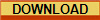Publication History: This article is based on Chapter 10 of "The Log Analysis Handbook" by E. R. Crain, P.Eng., published by Pennwell Books 1986  Updated 2004. This webpage version is the copyrighted intellectual property of the author. Do not copy or distribute in any form without explicit permission.Permeability from Porosity

This simple regression of core porosity versus core permeability:
1: PERMp = 10 ^ (HPERM * PHIe + JPERM)
2: IF PERMp > 20000
3: THEN PERMp = 20000

Where:
JPERM = permeability constant (fractional)
HPERM = porosity exponent (fractional)
PERMp = calculated permeability (millidarcies)
PHIe = effective porosity (fractional)COMMENTS:
Because a poor choice of HPERM could create very large values of permeability, the computation is usually constrained to prevent unreasonable answers. Results have been very good in low and high porosity when calibrated to core.

You can fit a regression line to anything - look at the graph and check the R-squared to confirm that the regression line is rational.RECOMMENDED PARAMETERS:
ROCK TYPE                HPERM            JPERM
Carbonates
chalky                         10 - 20               -2.5
fine sucrosic or
intercrystalline            20 - 30                -2.5
coarse sucrosic or
intercrystalline            25 - 50               -2.5
small vuggy                 30 - 100             -2.5
large vuggy                  50 - 200             -2.5
fractures 200 -                  300                 -3.0

Sandstones
very fine grains            10 - 20                -3.0
fine grains                    15 - 25                -3.0
medium grains             20 - 30                -3.0
large grains                  25 - 50                -3.0
conglomerate               20 - 50                 -3.0
unconsolidated             20 - 50                 -3.5
fractures                      200 - 300                -3.0Permeability from Porosity - example of a "good" regression line.NUMERICAL EXAMPLE
Assume data from Classic Example Sand B.
PHIe = 0.30
SWir = Sw = 0.25

1. Wylie-Rose formula / Morris-Biggs parameters:
CPERM = 62500
DPERM = 6.0
EPERM = 2.0
PERMw = 62500 * (0.30 ^ 6) / (0.25 ^ 2) = 730 md

2. Wylie-Rose formula / Timur parameters:
CPERM = 3400
DPERM = 4.4
EPERM = 2.0
PERMw = 3400 * (0.30 ^ 4.4) / (0.25 ^ 2) = 272 mdPermeability from Formation Factor
Since Formation Factor (F) is a function of porosity, F is also a predictor of permeability:
4: F = A / (PHIe ^ M)
5: PERMff = FPERM / (F ^ GPERM)

Where:
A = tortuosity exponent (fractional)
FPERM = permeability constant (fractional)
GPERM = porosity exponent (fractional)
F = formation factor (fractional)
M = cementation exponent
PERMf = calculated permeability (millidarcies)
PHIe = effective porosity (fractional)COMMENTS:
This formula has not been as successful as the Wylie - Rose approach.RECOMMENDED PARAMETERS:
ROCK TYPE               FPERM              GPERM
Sandstone             7.0 * 10 ^ 6              4.5
Limestone             4.0 * 10 ^ 6              3.5

Data from individual core studies varies considerably from these average values.NUMERICAL EXAMPLE
Assume data from Classic Example Sand B.
PHIe = 0.30
A = 0.62
M = 2.15Formation factor method:
FPERM = 7.0 * 10 ^ 6
GPERM = 4.50
F = 0.62 / (0.30 ^ 2.15) = 8.25
PERMf = 7.0 * 10 ^ 6 / (8.25 ^ 4.5) = 526 md"META/PERM"  Compare Permeability Calculated from Various MethodsPage Views ---- Since 01 Jan 2015